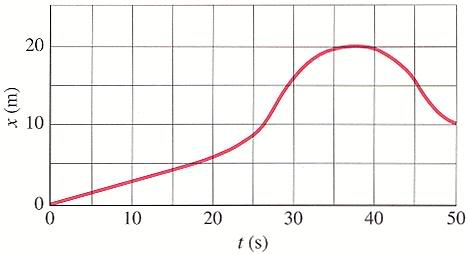# Negative acceleration and instantaneous velocity on an object.

CaptFormal

## Homework Statement

The position of an object along a straight tunnel as a function of time is plotted below:1. Are there any time intervals in which the object has a negative acceleration? If so, list them and explain how you know.

2. Calculate the instantaneous velocity at t=20s and t-45s.

N/A

## The Attempt at a Solution

So, for the first question I stated that at 30s to 37s the object's velocity is decreasing, thus the acceleration is negative. However, I was told that this is only part of the interval. What am I missing?

For the second question I did the following calculations:

at 20s = (6 m)/(20s) = 0.3 m/s
at 45s = (16m)/(45s) = 0.36 m/s

I did get the instantaneous velocity at 20s correct but not the instantaneous velocity at 45 s. Where am I going wrong?

Mindscrape
I would say that the velocity is decreasing from about 27 seconds (at the inflection point) to about 46s (at the other inflection point). Draw tangent lines to the graph, and note the parts where those slopes are getting smaller and smaller (the slope of the slopes).

I think you must be close for the one at 45 seconds. It should be around what you got, I'd say more around .43m/s though it's an estimate when you are using a graph rather than the function.

Homework Helper

## Homework Statement

So, for the first question I stated that at 30s to 37s the object's velocity is decreasing, thus the acceleration is negative. However, I was told that this is only part of the interval. What am I missing?

The velocity is decreasing up until 45 s. Remember that velocity can be negative, so if velocity goes from -1 m/s to -2 m/s, it's decreasing.
For the second question I did the following calculations:

at 20s = (6 m)/(20s) = 0.3 m/s
at 45s = (16m)/(45s) = 0.36 m/s

I did get the instantaneous velocity at 20s correct but not the instantaneous velocity at 45 s. Where am I going wrong?

The velocity should be negative, not positive. Also, you're calculating average velocity. The question is looking for instantaneous velocity, which is the slope of the tangent of the graph.